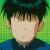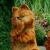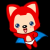## 请教一个JQuery hover的方法

jQuery(".aa#p1").hover(function(){

jQuery(".ga#p1").show();
},function(){
jQuery(".ga#p1").hide();

});

jQuery(".aa#p2").hover(function(){

jQuery(".ga#p2").show();
},function(){
jQuery(".ga#p2").hide();

});

jQuery(".aa#p3").hover(function(){

jQuery(".ga#p3").show();
},function(){
jQuery(".ga#p3").hide();

});

0```\$(function(){
\$("a[class^='aa#p']").each(function(index){
\$(this).hover(function(){

});
});
});```

```\$(function(){
\$("a[class^='aa#p']").hover(function(){
\$(this).next().show();
});
});```

0```for(var i=0;i<=30;i++){
\$(".aa#p"+i).hover(function(){
\$(".ga#p"+i).show();
},function(){
\$(".ga#p"+i).hide();
});
}```

0#### 引用来自“风吹屁屁凉”的评论

```\$(function(){
\$("a[class^='aa#p']").each(function(index){
\$(this).hover(function(){

});
});
});```

```\$(function(){
\$("a[class^='aa#p']").hover(function(){
\$(this).next().show();
});
});```